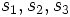# 2-subnormal implies join-transitively subnormal

Jump to: navigation, search
This article gives the statement and possibly, proof, of an implication relation between two subgroup properties. That is, it states that every subgroup satisfying the first subgroup property (i.e., 2-subnormal subgroup) must also satisfy the second subgroup property (i.e., join-transitively subnormal subgroup)
View all subgroup property implications | View all subgroup property non-implications
Get more facts about 2-subnormal subgroup|Get more facts about join-transitively subnormal subgroup
This article gives the statement and possibly, proof, of an implication relation between two subgroup properties. That is, it states that every subgroup satisfying the first subgroup property (i.e., 2-subnormal subgroup) must also satisfy the second subgroup property (i.e., linear-bound join-transitively subnormal subgroup)
View all subgroup property implications | View all subgroup property non-implications
Get more facts about 2-subnormal subgroup|Get more facts about linear-bound join-transitively subnormal subgroup

## Statement

### Statement with symbols

Suppose$H, K \le G$ are subgroups such that$H$ is a 2-subnormal subgroup of$G$ and$K$ is a subnormal subgroup of$G$. Then, the join$\langle H, K \rangle$ is a subnormal subgroup of$G$, and its subnormal depth in$G$ is at most twice the subnormal depth of$K$.

## Facts used

1. 2-subnormality is conjugate-join-closed: A join of any collection of 2-subnormal subgroups that are conjugate to each other is again 2-subnormal.
2. Join of subnormal subgroups is subnormal iff their commutator is subnormal: Suppose$H, K$ are subnormal subgroups of a group$G$. Then, consider the subgroups$[H,K]$ (the commutator) of$H$ and$K$), the subgroup$H^K$ (the join of$H^k$ for all$k \in K$) and the subgroup$\langle H, K \rangle$. If any one of these is subnormal, so are the other two. Further, if$s_1, s_2, s_3$ denote respectively the subnormal depths of$[H,K], H^K, \langle H, K \rangle$, we have$s_3 \le s_2k$.

## Proof

This proof uses a tabular format for presentation. Provide feedback on tabular proof formats in a survey (opens in new window/tab) | Learn more about tabular proof formats|View all pages on facts with proofs in tabular format

Given: A group$G$, a$2$-subnormal subgroup$H$, a$k$-subnormal subgroup$K$.

To prove:$\langle H, K \rangle$ is a$2k$-subnormal subgroup of$G$.

Proof:

Step no. Assertion Facts used Given data used Previous steps used Explanation
1$H^K$, i.e., the join (subgroup generated) of conjugates of$H$ by elements of$K$, is a 2-subnormal subgroup of$G$ Fact (1)$H$ is 2-subnormal in$G$ --
2$\langle H, K \rangle$ is$2k$-subnormal Fact (2)$K$ is$k$-subnormal Step (1) [SHOW MORE]